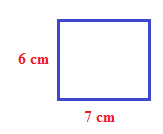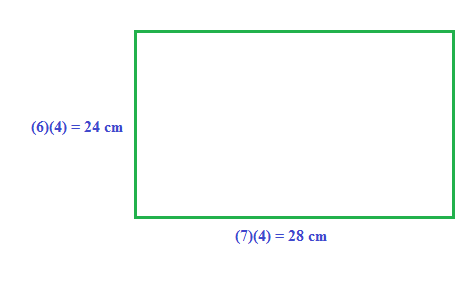### Home > CC2MN > Chapter 4 > Lesson 4.4.2 > Problem4-139

4-139.

The area of a rectangle is $42$ cm2. If the length and width each grow four times longer, what will the new area be?

Make an example of a rectangle with an area of $42$ cm2.
Since the area of a rectangle is (base)(height), choose a base and height whose product is $42$.  An example is shown.Multiply the base and height by $4$.Now find the area of the larger rectangle by multiplying the new base and the new height.